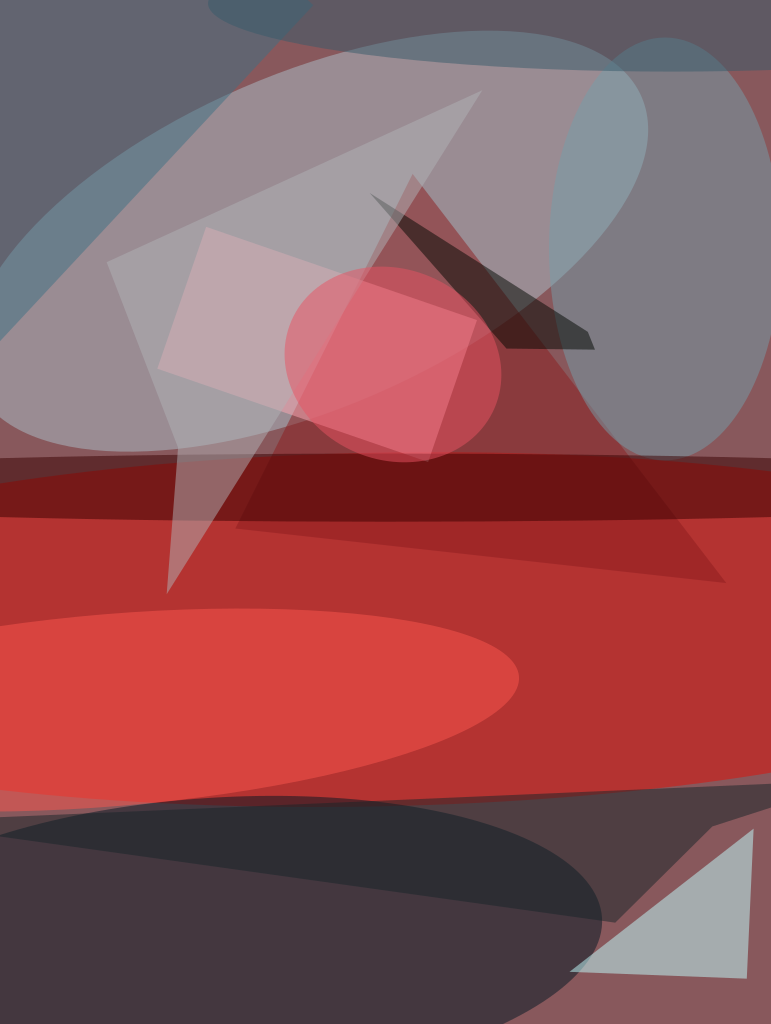Conventional Shack-Hartmann Wavefront Sensing requires a point source such as a star to perform wavefront sensing. This software allows one to conduct such sensing using an extended-scene or scene-based image. The software allows a Shack-Hartmann Wavefront Sensor (SH-WFS) to be used with an extended scene. It estimates the shift between the positions of a reference and test extended-scene sub-images or cells.

It runs a series of six steps:

1. It determines the centers of all of the sub-images, and stores these x and y positions in a [2×Ncell] matrix. Usually, the centroids of the point-source spot-images measured in the same system can serve this purpose.
2. It chooses an N×N-pixels subaperture, S(x,y), within each sub-image with the x and y positions determined in step 1 as its center, where N is preferentially a power of two for this algorithm (e.g., N=32). Such a subaperture is referred to as a test cell. It also chooses one M×M-pixels reference cell, r(x,y), preferably near the center of the whole extended scene image, where M<N is preferentially also a power of two (e.g., M=16).
3. It calculates the cross-correlation (CC) of r(x,y) and s(x,y), where s(x,y) is the central M×M-pixels portion of S(x,y), using FFT (fast Fourier transform). It determines the location of the CC-peak by fitting a quadratic curve to three points near and including the CC-peak in the xdirection, and doing the same in the y-direction. This is done analytically since there are three data for three unknown parameters in such a fit.
4. It shifts the test cell S(x,y)by the amount determined in the previous step to match it with the reference cell.
5. It repeats Steps 3 and 4 in an iteration loop, while accumulating the sub-image offsets dx and dy between the test and the reference cells, until a maximum iteration number is reached or the incremental change in sub-image offset becomes smaller than a pre-determined tolerance.
6. It repeats Steps 2 to 5 for all of the test cells.

This work was done by Erkin Sidick of Caltech for NASA’s Jet Propulsion Laboratory.

The software used in this innovation is available for commercial licensing. Please contact Dan Broderick at This email address is being protected from spambots. You need JavaScript enabled to view it.. Refer to NPO-48003.

##### This Brief includes a Technical Support Package (TSP).###### Adaptive Periodic-Correlation Algorithm for Shack-Hartmann Wavefront Sensing